# NCERT Solutions for Class 3 Maths Can we Share## myCBSEguide App

CBSE, NCERT, JEE Main, NEET-UG, NDA, Exam Papers, Question Bank, NCERT Solutions, Exemplars, Revision Notes, Free Videos, MCQ Tests & more.

NCERT solutions for Class 3 Mathematics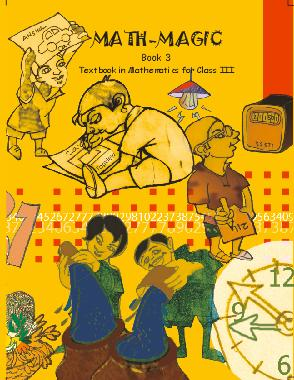## NCERT Solutions for Class 3 Maths Can we Share

CHAPTER 12 Class 3 Maths Can we Share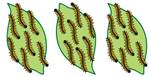### (a)There are ___ caterpillars.

Ans. 21 caterpillars.

(b) There are in ___ groups.

Ans. 3 groups.

(c) There are ___ caterpillars in each group.

Ans. (c) 7 caterpillars.

### 2.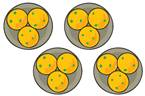(b) They are in ___ groups.

Ans. 4 groups.

(c) There are __ laddoos in each group.

### 3. Mummy bird brings 12 grains. How to distribute equally? Mummy bird starts by giving 1 grain to each baby. Then Mummy bird gives one grain to each baby. Each baby has got 2 grains now. How many grains are left?

Ans. 4 (As 12 – 4 – 4 = 4)

### 4. Now draw the jalebis on the plates below, so the plates, so that each plate has the same number of jalebis.

Ans.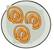Ans. 9 jalebis.

Ans. 3 jalebis.

### 7. Discuss in the class how you found the answer.

Ans. Take total jalebis 1 + 5 + 3 = 9

Now we want to place equally in 3 plates as 9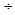3 = 3. So, put 3 jalebis in each plate.

### 8. If there are 60 bananas and two monkeys, how many will each monkey get?

Ans. 602= 30 bananas.

### 9. Five friends found RS. 100. If they share it equally, how much will each get?

Ans. Rs, 1005 = Rs. 20

### 10. Hari Prashad has 30 metres of rope. He distributes it equally among his three children. Each child get ___ metres of rope.

Ans. 303 = 10 metres of rope.

### 11. If there is 36 metres of rope, how much of rope will each child gets.

Ans. 363 = 12 metres.

### 12. If there is 60 metres of rope, how much will each child get?

Ans. 603 = 20.

### 13. Minku puts her 15 laddoos equally into 5 boxes.

(a) How many ladoos will there be in each box?

Ans. There will be 3 laddoos in each box. 155 = 3.

(b) If she uses only 3 boxes, how many laddoos will there in each box?

Ans. There will be 5 laddoos in each box. 153 = 5.

### 14. Share 25 bananas among 5 monkeys. How many bananas for each monkey?

Ans. 255 = 5.

### 15. Share 12 balloons among 3 boys. How many balloons for each boy?

Ans. 123 =4. Every boy has 4 balloons.

### 16. There are 21 candles. Put them equally in 3 boxes. How many candles are there in each box?

Ans. 213 = 7 candles.

### 17. There are 18 socks. How many girls can wear these socks?

Ans. 9 girls can wear these socks. 182 = 9.

### 18. Raj has 36 minutes to make rotis. One roti takes 3 minutes. How many this can be make in this time?

Ans. He can make 12 rotis. 363 = 12.

### 19. These are 24 footmarks of goats. So how many goats were there?

Ans. There are 6 goats, 244= 6.

### 20. Some girls are playing a game with both their hands. The girls who are playing have 60 fingers altogether. How many girls are playing this game?

Ans. There are 10 girls. 6010 = 6.

### 21. Lakshmi has 27 potatoes to sell. There men came and bought equal amount of potatoes.

Ans. 273 = 9 kg of potatoes.

### 22. In how many jumps will the frog reach 30?

Ans. 302 = 15 jumps.

### 23. In how many jumps will the squirrel reach 27?

Ans. 273 = 9 jumps.

### 24. Which number will the kangaroo reach in two jumps.

Ans. 30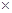2 = 60.

### 25. Who all will meet at the number 18?

Ans. Rabbit, Horse.

Ans. No.

### 27. How many jumps of the rabbit equal one jump of the horse?

Ans. 155 = 3 jumps.

### 28. How many jumps of the horse equals two jumps of the kangaroo?

Ans. In two jumps of the kangaroo reach = 302 = 60.

Number of jumps taken by horse to reach 60 = 6015 = 4.

### 29. Which is the smallest number where the frog and the squirrel will meet?

Ans. The smallest number where the frog and the squirrel will meet is 23 = 6.

## NCERT solutions for Class 3 Mathematics Chapter 12 Can we Share

NCERT Solutions Class 3 Maths Can we Share PDF (Download) Free from myCBSEguide app and myCBSEguide website. Ncert solution class 3 Maths includes text book solutions from Class 3 Maths Book . NCERT Solutions for CBSE Class  Maths have total 14 chapters. 3 Maths NCERT Solutions in PDF for free Download on our website. Ncert Maths class 3 solutions PDF and solutions with latest modifications and as per the latest CBSE syllabus are only available in myCBSEguide.

## CBSE app for Students

To download NCERT Solutions for Class 3 EVS Hindi English, Maths do check myCBSEguide app or website. myCBSEguide provides sample papers with solution, test papers for chapter-wise practice, NCERT solutions, NCERT Exemplar solutions, quick revision notes for ready reference, CBSE guess papers and CBSE important question papers. Sample Paper all are made available through the best app for CBSE students and myCBSEguide website.